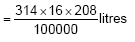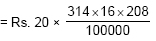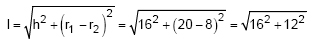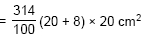Newbie

# A container, opened from the top and made up of a metal sheet, is in the form of a frustum of a cone of height 16 cm with radii of its lower and upper ends as 8 cm and 20 cm, respectively. Find the cost of the milk which can completely fill the container, at the rate of Rs. 20 per litre. Also find the cost of metal sheet used to make the container, if it costs Rs. 8 per 100 cm2.Q.4

• 0

Today i am solving the important question of ncert of class 10 chapter surface areas and volumes of exercise  13.4 question number 4 . Please help me to solve the tricky question of this question . Give me the best solution of this question. A container, opened from the top and made up of a metal sheet, is in the form of a frustum of a cone of height 16 cm with radii of its lower and upper ends as 8 cm and 20 cm, respectively. Find the cost of the milk which can completely fill the container, at the rate of Rs. 20 per litre. Also find the cost of metal sheet used to make the container, if it costs Rs. 8 per 100 cm2.

Share

1. Given,

r1 = 20 cm,

r2 = 8 cm and

h = 16 cm

∴ Volume of the frustum = (⅓)×π×h(r12+r22+r1r2)It is given that the rate of milk = Rs. 20/litre

So, Cost of milk = 20×volume of the frustum= Rs. 209

Now, slant height will beSo, CSA of the container = π(r1+r2)×l= 1758.4 cm2

Hence, the total metal that would be required to make container will be = 1758.4 + (Area of bottom circle)

= 1758.4+201 = 1959.4 cm2

∴ Total cost of metal = Rs. (8/100) × 1959.4 = Rs. 157

• 0